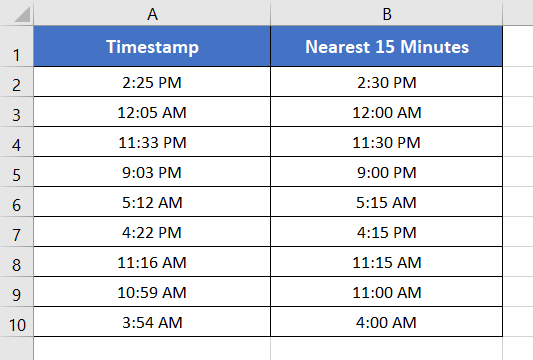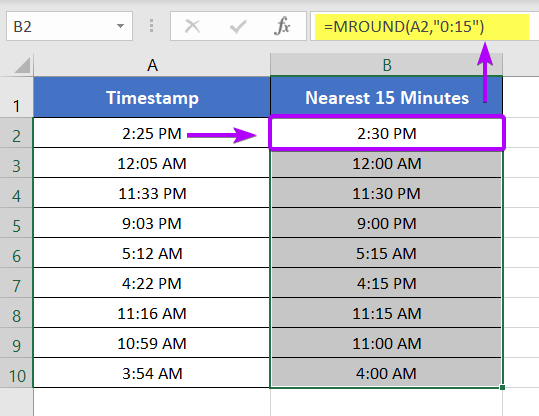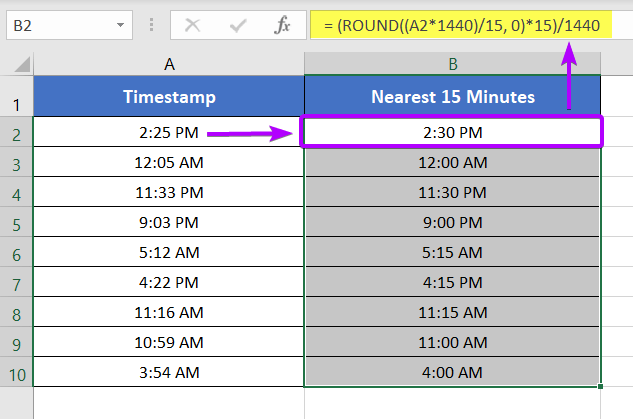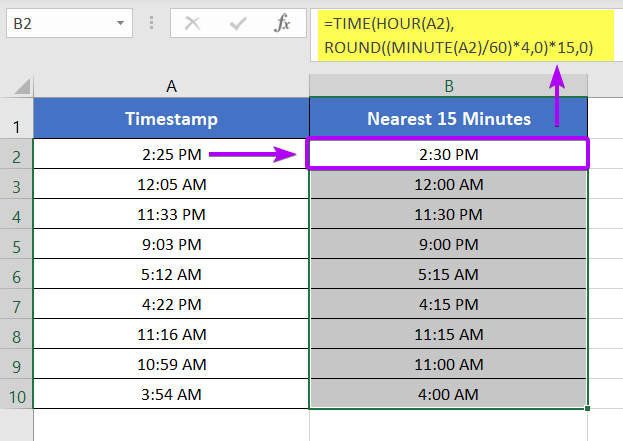# [3 Formulas] Round Off Time to Nearest Multiple of 15 Minutes in Excel

Microsoft Excel has multiple functions to round off numbers. Among them, the ROUND, MROUD, FLOOR, CEILING, etc. are some of the functions. In this tutorial, I will show you how to round off time to the nearest multiple of 15 minutes in Excel using the ROUND and MROUND functions.

Here’s the Excel file that I’ve used throughout the whole blog. You can download it from the following link:

### Introduction to the Dataset

In the column, Timestamp, I have some random timestamps. In the column, Nearest 15 Minutes, I will use formulas to round all the timestamps to the nearest multiple of 15 minutes.## Easiest Way to Round Off Time to the Nearest Multiple of 15 Minutes

Excel has a dedicated function to round off numbers to a certain multiple. The name of the function is MROUND. Now I will use the MROUND function to round off the timestamps to the nearest multiple of 15 minutes in Excel.

Syntax

`=MROUND(number, multiple)`

Formula

`=MROUND(A2,"0:15")`

Usage Guide

Step_1: Type the formula in cell B2.

Step_2: Press ENTER.

Step_3: Copy down the formula to cell B10 by dragging the lower-right corner of cell B2.

Final Result

The MROUND function rounds off all the timestamps to their corresponding nearest multiple of 15 minutes.

For example, the timestamp 2:25 PM has two bracket timestamps that are multiple of 15 minutes. They are 2:15 PM and 2:30 PM. The timestamp 2:30 PM is closer to 2:25 PM than that of 2.15 PM.

Thus, the formula has returned 2:30 PM in cell B2 instead of 2:15 PM.Formula Explanation

According to the syntax of the MROUND function, cell A2 is the number. And “0:15” is multiple. The multiple specifies that the formula must return the nearest multiple of 15 minutes of the specified number i.e. 2:25 PM.

If you mention any other multiple other than 0:15, the formula will return the corresponding nearest multiple of the specified multiple.

### Alternative Way #1: Using ROUND Function

You can also use the ROUND function instead of the MROUND function. But you need to add some extra weights in order to calculate the nearest multiple of 15 minutes. Now let me show you how to round off time to the nearest multiple of 15 minutes.

Syntax

`=ROUND(number, num_digits)`

Formula

`=(ROUND((A2*1440)/15,0)*15)/1440`

Usage Guide

Step_1: Type the formula in cell B2.

Step_2: Press ENTER.

Step_3: Copy down the formula to cell B10 by dragging the lower-right corner of cell B2.

Final Result

The above formula rounds off all the timestamps to their corresponding nearest multiple of 15 minutes.

For example, the timestamp 2:25 PM has two bracket timestamps that are multiple of 15 minutes. They are 2:15 PM and 2:30 PM. The timestamp 2:30 PM is closer to 2:25 PM than that of 2.15 PM.

Thus, the formula has returned 2:30 PM in cell B2 instead of 2:15 PM.Formula Explanation

The formula used above is (ROUND((A2*1440)/15, 0)*15)/1440. We can rewrite the formula as (ROUND((A2*24*60)/15, 0)*15)/(24*+60).

First, the time is converted to minutes to the multiple of 15 minutes. This is done by multiplying the time with 1440 and then dividing it by 15. Then the fraction is rounded off using the ROUND function. In the formula, 0 specifies that there will be no decimal places after the decimal point. Thus the ROUND function returns solid rounded minutes of the time in cell A2.

Finally, the rounded minutes are multiplied by 15 again and divided by 1440 to get back to the original time format again.

### Alternative Way #2: Using TIME, HOUR, MINUTE, & ROUND Functions

Finally, I will use a combination of the TIME, HOUR, MINUTE, & ROUND functions. This combination will return the rounded-off times to their nearest multiple of 15 minutes.

Syntax

`=TIME(HOUR(serial_number),ROUND((MINUTE(serial_numbe)/60)*4,0)*15,0)`

Formula

`=TIME(HOUR(A2),ROUND((MINUTE(A2)/60)*4,0)*15,0)`

Usage Guide

Step_1: Type the formula in cell B2.

Step_2: Press ENTER.

Step_3: Copy down the formula to cell B10 by dragging the lower-right corner of cell B2.

Final Result

The formula converts all the timestamps to their nearest multiple of 15 minutes. To return the nearest multiple of the timestamps, sometimes the formula rounded up the times. Sometimes the formula is also rounded down the timestamps to calculate the nearest multiple of 15 minutes.Formula Explanation

Here, the main formula is made up of the TIME function.

The syntax of the TIME function is:

`=TIME(hour, minute, second)`

The main idea behind the formula is to work with hours, minutes, and seconds separately.

• HOUR(A2) returns 14. But it is converted to 2 as the cell format is set to 12-Hour time format.
• (MINUTE(A2)/60)*4 converts the minute part of the time in cell A2 to the multiple of 15 minutes.
• ROUND((MINUTE(A2)/60)*4,0) rounds off the minutes returned by (MINUTE(A2)/60)*4 to the nearest integer value.
• The 0 at the end of the formula specifies that the second part is not considered in the formula.
• Finally, the TIME function returns the converted time of cell A2 into the hour, minute, and second format.

## Conclusion

So, I have discussed 3 effective methods to round off time to the nearest multiple of 15 minutes in Excel. I expect you’ve found this article helpful. You can read more Excel articles from our website’s Blog page. Have a great workday!

(Visited 139 times, 1 visits today)